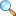# Chapter 11: The Laplace Transform

Notes of the book Mathematical Method written by S.M. Yusuf, A. Majeed and M. Amin. This book is published by Ilmi Kitab Khana, Lahore - PAKISTAN. Solutions of Chapter 11: The Laplace Transform are given here in pdf form.

Let $f$ be a real valued piecewise continuous function defined on $[0,\infty)$. The Laplace transform of $f$, denoted by $\mathcal{L}(f)$, is the function $F$ defined by $F(s)=\int_0^{\infty} e^{-st} f(t) dt,$ provided the above improper integral converges. We have $F=\mathcal{L}(f)$.

Here is the list of other contents given in this chapter.

• The Laplace transform
• Properties of the Laplace transform
• Exercise 11.1
• Inverse Laplace transform
• Convolution
• Exercise 11.2
• Solution of initial value problem
• Exercise 11.3

#### Notes by Umer Asghar

• Laplace Transformation, Exercise 11.1 |View online | Download PDF (1419KB)
• Inverse Laplace Transformation, Ex 11.2 |View online | Download PDF (1270KB)

#### Notes by Prof Rizwan Saleem

These notes are written by Muhammad Zahid Iqbal based on the lectures of Prof. Rizwan Saleem
• Chapter 11: The Laplace Transoformation |View online | Download PDF (8894KB)• bsc/notes_of_mathematical_method/ch11_the_laplace_transform